Learning Library

Multiplication Mania! Ten 3rd Grade Multiplication Worksheets

Help your third grader master her times tables with this collection of multiplication worksheets.

Click on a worksheet in the set below to see more info or download the PDF.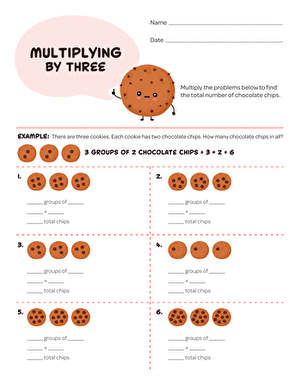Multiplying by 3

Practice multiplying by 3 with this sweet, cookie-themed worksheet. Multiplication is a lot more fun when ice cream is involved!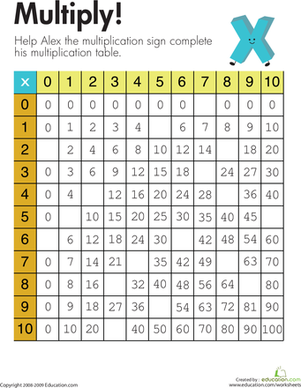Alex's Multiplication Table

Kids fill in the missing spaces of the multiplication table to complete this second and third grade math worksheet.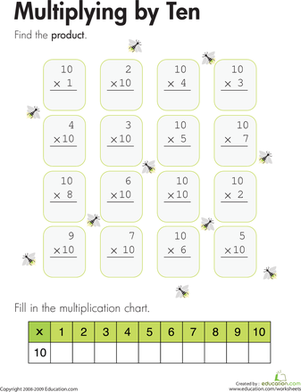Multiplying by Ten

Kids completing this third grade math worksheet multiply by 10 to solve each equation and also fill in a multiplication chart for the number 10.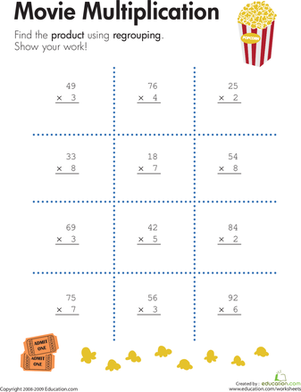2-Digit by 1-Digit Multiplication

Kids solve problems involving multiplication of 2-digit numbers by 1-digit numbers using regrouping on this third grade math worksheet.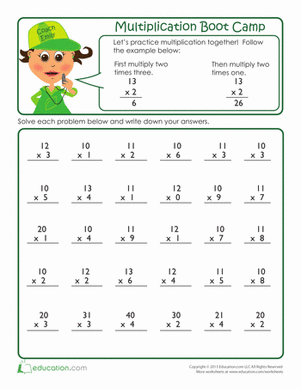Multiplication Boot Camp

Put your multiplication skills to the test with this practice drill focusing on the 10, 11, and 12 times tables.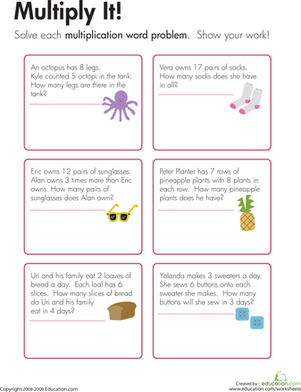Multiplication Word Problems: Multiply It!

Kids use details from the word problems on this third grade math worksheet to construct and solve multiplication problems.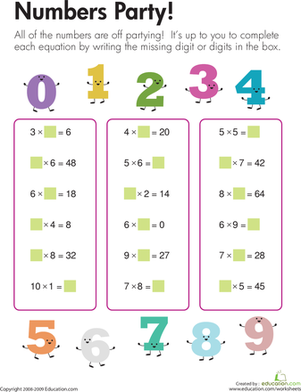Multiplication Fun

Have some multiplication fun! The numbers in the equations are missing because they're off at a numbers party. Fill in the missing numbers.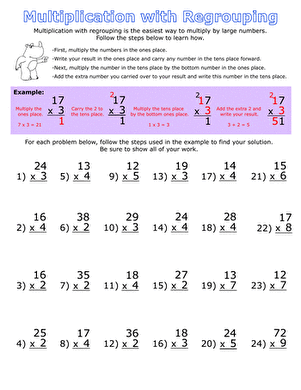Multiplication with Regrouping

Offering over 40 regrouping problems, this worksheet is a great way to practice your multiplication skills.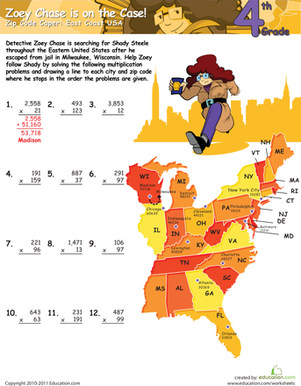Multiplication Mischief: East Coast USA

Enlist your fourth grader's investigative math skills to find the suspect responsible for some multiplication mischief!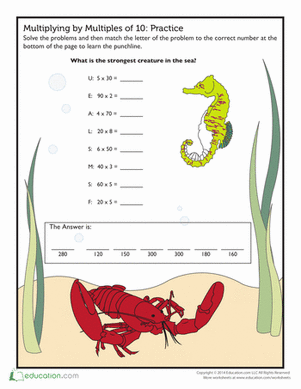Silly Seahorse Multiplication Practice

This worksheet is a great way for your kid to practice multiplying by multiples of ten. Once she's done she'll get to giggle at the seahorse's silly joke.

Want to download this whole set as a single PDF?

Create new collection

0

New Collection>

0 items

What could we do to improve Education.com?

Please note: Use the Contact Us link at the bottom of our website for account-specific questions or issues.

What would make you love Education.com?